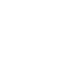# ACT & SAT Question of the Day: May 28, 2014

If you are reading this in an email you received from me, do not click the link to sat.collegeboard.org below. Use the link to my website that is farther down on the email.

http://sat.collegeboard.org/practice/sat-question-of-the-day?questionId=20140528&oq=1 (This link takes you to today’s question. If you use my archive, you will see the question related to my SAT explanation for that date.)

Reading this blog is 10% about learning how to answer today’s questions and 90% learning how to apply strategies and analyze questions you may see on test day.

The answer is B.  The size of x is irrelevant and only included as part of the question to confuse you.  After all, when you calculate the area of a cube, you square the length of the side and multiply by 6 and when you calculate the volumes of a cube you cube the length of the side.  Because x is squared in the word problem to get the surface area, the answer has to include an x cubed.

Each side of the cube is 54/6 because there are 6 sides which is 9.  The square root of 9 is 3; so, each side of the cube is 3.  3 cubed is 27–Answer B.

Did you notice that 27 is half of 54?  Yes, it is.  However, don’t think that the volume is always going to be half the surface area!  For example, if the side were 2, the surface area would be 24–6(2×2) = 24 and the volume would be 2 cubed which is 8.  8 isn’t half of 24!  There is no pattern of halving the surface area to get the volume.

Let’s see what the ACT folks have for us today.

The answer is D.  Oxygen is the only chemical that is constant from the depth of 10cm and beyond.

What can you learn about the ACT Science Test from this question?  It’s a good example of some fundamental truths about the test.  First, don’t review science; you’ll never predict the right content.  Second, the questions are answered in the passage.  (I’ve only seen two exceptions to that statement in over 20 years!)  Third, practicing reading and interpreting charts and graphs is critical to your success.  Fourth, speed is very important.  Most students complain about lack of time when it comes to finishing the ACT Science Test.

QotD Words of “Wiz-dom”:

You cannot cram for the SAT or ACT.  If you are serious about raising your score, you have to improve your test-taking skills, not simply review content.  My complete programs take about 50 hours to complete.  That is 15 hours watching videos (or reading my website which takes longer) and 35 hours of practice if you need to work on all the sections of the two tests.  Of course, raising your score on one section of one test will take less time.  For example, you can improve your reading test-taking skills in only a few hours.  That is the section of the test that you can see the greatest score increase in the least amount of time.

This is all true because the tests are about a skill set, not simply knowledge.  Developing skills takes practice, not memorization.  You can memorize my strategies in a very short period of time but unless you practice them, you won’t see nearly the score increase you’ll experience if you spend some time practicing.

Bob Alexander, the “SAT and ACT Wizard”